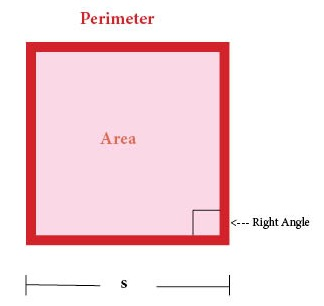# Measurement

1 foot = 12 inches

1 meter = 100 centimeters

# Parallelogram

Parallelogram = Flat Four-Sided Shape in which Opposite Sides are Parallel

# Triangle

Triangle = Flat Shape with Three Sides# Rectangle

Rectangle = Flat Shape with Four Sides (with four right angles)

# Square

Square = Flat Shape with Four Equal Sides (with four right angles)